Important Questions: Congruence of Triangles

# Important Questions: Congruence of Triangles

Test Description

## 20 Questions MCQ Test Online MCQ Tests for Class 7 | Important Questions: Congruence of Triangles

Important Questions: Congruence of Triangles for Class 7 2022 is part of Online MCQ Tests for Class 7 preparation. The Important Questions: Congruence of Triangles questions and answers have been prepared according to the Class 7 exam syllabus.The Important Questions: Congruence of Triangles MCQs are made for Class 7 2022 Exam. Find important definitions, questions, notes, meanings, examples, exercises, MCQs and online tests for Important Questions: Congruence of Triangles below.
Solutions of Important Questions: Congruence of Triangles questions in English are available as part of our Online MCQ Tests for Class 7 for Class 7 & Important Questions: Congruence of Triangles solutions in Hindi for Online MCQ Tests for Class 7 course. Download more important topics, notes, lectures and mock test series for Class 7 Exam by signing up for free. Attempt Important Questions: Congruence of Triangles | 20 questions in 20 minutes | Mock test for Class 7 preparation | Free important questions MCQ to study Online MCQ Tests for Class 7 for Class 7 Exam | Download free PDF with solutions
 1 Crore+ students have signed up on EduRev. Have you?
Important Questions: Congruence of Triangles - Question 1

### In ΔABC,AB = AC and AD is ⊥ to BC. State the property by which ΔADB = ΔADC.

Detailed Solution for Important Questions: Congruence of Triangles - Question 1

According to the question, the triangles formed are right angled triangles, as shown in the figure.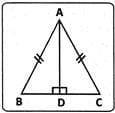Important Questions: Congruence of Triangles - Question 2

### Two students drew a line segment each. What is the condition for them to be congruent?

Detailed Solution for Important Questions: Congruence of Triangles - Question 2

Line segments of equal length are congruent.

Important Questions: Congruence of Triangles - Question 3

### In the given figure, if AD = BC and AD||BC,which of the following is true?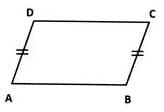Detailed Solution for Important Questions: Congruence of Triangles - Question 3

In the given figure,AD = BC,AC = AC,  and ∠DAC = ∠BCA
By S.A.S. theorem ΔABC≅ΔCDA
So, AB=DC.
(Corresponding parts of congruent triangles.)

Important Questions: Congruence of Triangles - Question 4

In ΔPQR and ΔXYZ, ∠P = 50o, XY = PQ, and XZ = PR. By which property are ΔXYZ and ΔPQR congruent?

Detailed Solution for Important Questions: Congruence of Triangles - Question 4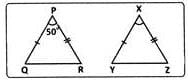In ΔPQR and ΔXYZ. ∠P = ∠X = 50o PQ = XY  and PR  =XZ.
∴ Δ PQR ≅ Δ XYZ (S.A.S. property)

Important Questions: Congruence of Triangles - Question 5

A: Two triangles are said to be congruent if two sides and an angle of one triangle are respectively equal to the two sides and an angle of the other.
R: Two triangles are congruent if two sides and the included angle of one are equal to the corresponding two sides and included angle of the other.
Given A & R, which of the following statements is correct?

Detailed Solution for Important Questions: Congruence of Triangles - Question 5

Including angle means the angle formed by two lines. This means that two sides of one triangle which are equal to two sides of other triangle have an including angle and only that angle should be equal.

Important Questions: Congruence of Triangles - Question 6

Two triangles, A PQR and ADEF are of the same size and shape. What can we conclude about them?

Detailed Solution for Important Questions: Congruence of Triangles - Question 6

Two triangles are congruent when they are of the same shape and size , if any one of them is made to superpose on the other , it will cover exactly .Since both are equal , they cannot be smaller or larger.

Important Questions: Congruence of Triangles - Question 7

Which of the following examines the congruence of plane figures?

Important Questions: Congruence of Triangles - Question 8

Which of the following is a pair of congruent figures?

Important Questions: Congruence of Triangles - Question 9

In ΔABC and ΔPQR, AB=x cm, BC=y cmand CA=z cm. What are the measures of sides PQ, QR and RP of ΔPQR if ΔABC ≅ ΔABC ≅ ΔPQR?

Detailed Solution for Important Questions: Congruence of Triangles - Question 9

Corresponding parts of congruent triangles are equal.
So, PQ = AB = x cm, QR = BC = y cm and RP = CA = z cm.

Important Questions: Congruence of Triangles - Question 10

In the given figure, AD = CD and AB = CB.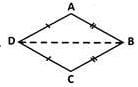What are the three pairs of equal parts?

Detailed Solution for Important Questions: Congruence of Triangles - Question 10

Corresponding sides in ΔABD and ΔCBD.

Important Questions: Congruence of Triangles - Question 11

In the given figure, AD = CD and AB = CB.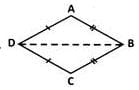Which of the following is the correct conclusion?

Detailed Solution for Important Questions: Congruence of Triangles - Question 11

Since ΔABD  and  ΔCBD  are  congruent, ∠ADB=∠CDB
(Corresponding parts of congruent triangles).⇒ BD bisects ∠ADC

Important Questions: Congruence of Triangles - Question 12

In the given figure, AB=AC and AD is the bisector of ∠BAC.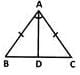Which among the following statements is true?

Detailed Solution for Important Questions: Congruence of Triangles - Question 12

We have AB=AC.Angles opposite to these sides will also be equal. So angles opposite to these sides are angle and angle c. So angle b and angle c are equal.

Important Questions: Congruence of Triangles - Question 13

In ΔABC and ΔDEF, AC = DF,AB = DE and BC=EF. By which property are ΔABC and ΔDEF congruent?

Important Questions: Congruence of Triangles - Question 14

For the triangles ΔART and ΔPEN given, if S.A.S. criterion should be used given ∠T = ∠N what are the respective measures of PN and RT?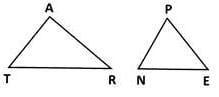Important Questions: Congruence of Triangles - Question 15

Which of the following is an example of A.S.A. criterion of congruency for two triangles ∠ACB and ∠DEF?

Important Questions: Congruence of Triangles - Question 16

In the figure given, AC = BD and ∠BAC = ∠CDB = 90o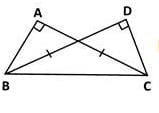If ΔABC = ΔDCB by R.H.S. property which of the following is required?

Detailed Solution for Important Questions: Congruence of Triangles - Question 16

BC is the hypotenuse of the given triangles. Whose measure must be known.

Important Questions: Congruence of Triangles - Question 17

Which of the following criterion does not exist?

Detailed Solution for Important Questions: Congruence of Triangles - Question 17

A.A.A. criterion does not exist.

Important Questions: Congruence of Triangles - Question 18

In two triangles, the three angles of one triangle are correspondingly equal to three angles of another triangle. Which of the following is a correct statement?

Detailed Solution for Important Questions: Congruence of Triangles - Question 18

If the three angles of a triangle are congruent to corresponding angles of the other, it is an enlarged copy of the triangle.

Important Questions: Congruence of Triangles - Question 19

ΔABC is congruent to ΔPQR under the correspondence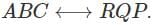Which part corresponds to PQ?

Detailed Solution for Important Questions: Congruence of Triangles - Question 19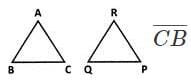corresponds to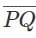Important Questions: Congruence of Triangles - Question 20

ΔABC is congruent to ΔPQR under the correspondence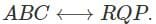Which part of corresponds to RP?

Detailed Solution for Important Questions: Congruence of Triangles - Question 20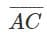corresponds to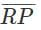[Note that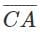corresponds to PR.]

## Online MCQ Tests for Class 7

178 tests
 Use Code STAYHOME200 and get INR 200 additional OFF Use Coupon Code
Information about Important Questions: Congruence of Triangles Page
In this test you can find the Exam questions for Important Questions: Congruence of Triangles solved & explained in the simplest way possible. Besides giving Questions and answers for Important Questions: Congruence of Triangles, EduRev gives you an ample number of Online tests for practice

178 tests